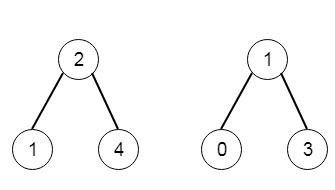# All Elements in Two Binary Search Trees in C++

C++Server Side ProgrammingProgramming

#### C in Depth: The Complete C Programming Guide for Beginners

45 Lectures 4.5 hours

#### Practical C++: Learn C++ Basics Step by Step

Most Popular

50 Lectures 4.5 hours

#### Master C and Embedded C Programming- Learn as you go

66 Lectures 5.5 hours

Suppose we have two binary search trees, we have to return a list of values, that has all elements present in these trees, and the list elements will be in ascending order. So if the trees are like −Then the output will be [0,1,1,2,3,4].

To solve this, we will follow these steps −

• Define an array called ans, define two stacks st1 and st2
• curr1 := root1 and curr2 := root2
• insert node root1 and all left nodes into st1, insert node root2 and all left nodes into st2
• while st1 is not empty or st2 is not empty
• if st1 is not empty, and (st2 is empty or top node value of st1 <= top node value of st2)
• temp := top of st1, delete node from st1
• insert value of temp into ans
• insert node right of temp and all left nodes of it into st1
• Otherwise −
• temp := top of st2, delete node from st2
• insert value of temp into ans
• insert node right of temp and all left nodes of it into st2
• return ans

## Example(C++)

Let us see the following implementation to get a better understanding −

Live Demo

#include <bits/stdc++.h>
using namespace std;
void print_vector(vector<auto> v){
cout << "[";
for(int i = 0; i<v.size(); i++){
cout << v[i] << ", ";
}
cout << "]"<<endl;
}
class TreeNode{
public:
int val;
TreeNode *left, *right;
TreeNode(int data){
val = data;
left = NULL;
right = NULL;
}
};
void insert(TreeNode **root, int val){
queue<TreeNode*> q;
q.push(*root);
while(q.size()){
TreeNode *temp = q.front();
q.pop();
if(!temp->left){
if(val != NULL)
temp->left = new TreeNode(val);
else
temp->left = new TreeNode(0);
return;
}
else{
q.push(temp->left);
}
if(!temp->right){
if(val != NULL)
temp->right = new TreeNode(val);
else
temp->right = new TreeNode(0);
return;
}
else{
q.push(temp->right);
}
}
}
TreeNode *make_tree(vector<int> v){
TreeNode *root = new TreeNode(v);
for(int i = 1; i<v.size(); i++){
insert(&root, v[i]);
}
return root;
}
class Solution {
public:
void pushLeft(stack <TreeNode*>& st, TreeNode* root){
TreeNode* curr = root;
while(curr){
st.push(curr);
curr = curr->left;
}
}
vector<int> getAllElements(TreeNode* root1, TreeNode* root2) {
vector <int> ans;
stack <TreeNode*> st1, st2;
TreeNode* curr1 = root1;
TreeNode* curr2 = root2;
pushLeft(st1, curr1);
pushLeft(st2, curr2);
while(!st1.empty() || !st2.empty()){
TreeNode* temp;
if(!st1.empty() && (st2.empty() || st1.top()->val <= st2.top()->val)){
temp = st1.top();
st1.pop();
ans.push_back(temp->val);
pushLeft(st1, temp->right);
}
else{
temp = st2.top();
st2.pop();
ans.push_back(temp->val);
pushLeft(st2, temp->right);
}
}
return ans;
}
};
main(){
vector<int> v = {2,1,4};
TreeNode *root1 = make_tree(v);
v = {1,0,3};
TreeNode *root2 = make_tree(v);
Solution ob;
print_vector(ob.getAllElements(root1, root2));
}

## Input

[2,1,4]
[1,0,3]

## Output

[0,1,1,2,3,4]